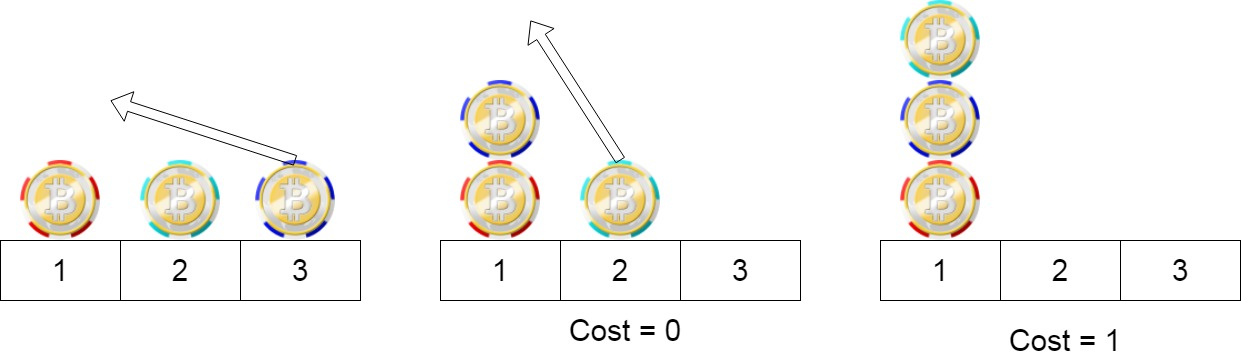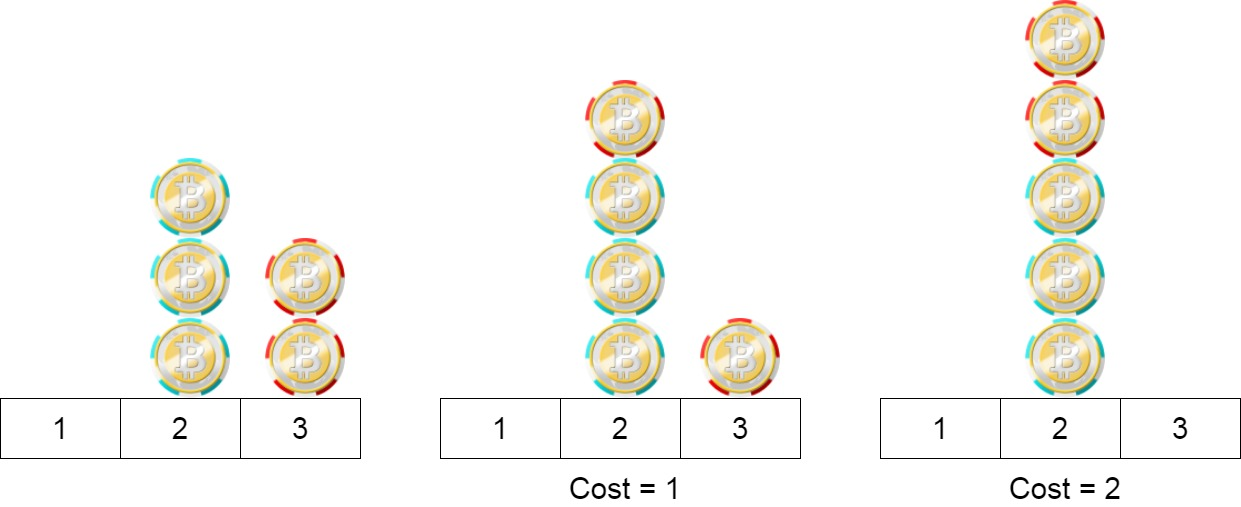1217. Minimum Cost to Move Chips to The Same Position

We have `n` chips, where the position of the `ith` chip is `position[i]`.

We need to move all the chips to the same position. In one step, we can change the position of the `ith` chip from `position[i]` to:

• `position[i] + 2` or `position[i] - 2` with `cost = 0`.
• `position[i] + 1` or `position[i] - 1` with `cost = 1`.

Return the minimum cost needed to move all the chips to the same position.

Example 1:```Input: position = [1,2,3]
Output: 1
Explanation: First step: Move the chip at position 3 to position 1 with cost = 0.
Second step: Move the chip at position 2 to position 1 with cost = 1.
Total cost is 1.
```

Example 2:```Input: position = [2,2,2,3,3]
Output: 2
Explanation: We can move the two chips at position  3 to position 2. Each move has cost = 1. The total cost = 2.
```

Example 3:

```Input: position = [1,1000000000]
Output: 1
```

Constraints:

• `1 <= position.length <= 100`
• `1 <= position[i] <= 10^9`

1217. Minimum Cost to Move Chips to The Same Position
``````struct Solution;

impl Solution {
fn min_cost_to_move_chips(chips: Vec<i32>) -> i32 {
let n = chips.len();
let mut a = 0;
let mut b = 0;
for i in 0..n {
if chips[i] % 2 == 0 {
a += 1;
} else {
b += 1;
}
}
i32::min(a, b)
}
}

#[test]
fn test() {
let chips = vec![1, 2, 3];
assert_eq!(Solution::min_cost_to_move_chips(chips), 1);
let chips = vec![2, 2, 2, 3, 3];
assert_eq!(Solution::min_cost_to_move_chips(chips), 2);
}
``````